# MeasurementA Common Language Mass Mass Mass The measure

• Slides: 23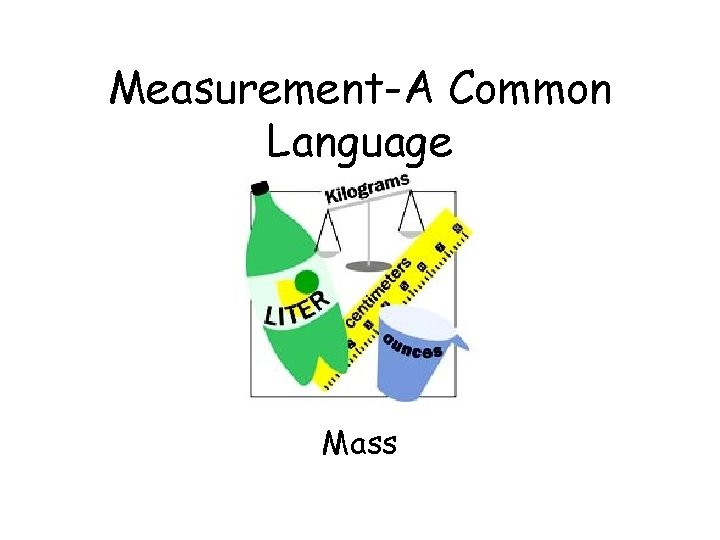Measurement-A Common Language MassMassMass • The measure of the amount of matter an object contains • Matter: The material that all objects and substances are made up of • Anything that has mass and takes up space is matterMass Units of Mass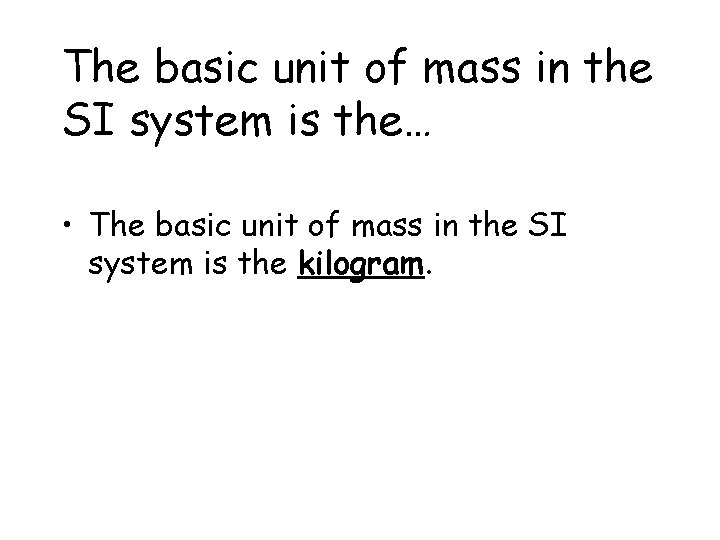The basic unit of mass in the SI system is the… • The basic unit of mass in the SI system is the kilogram.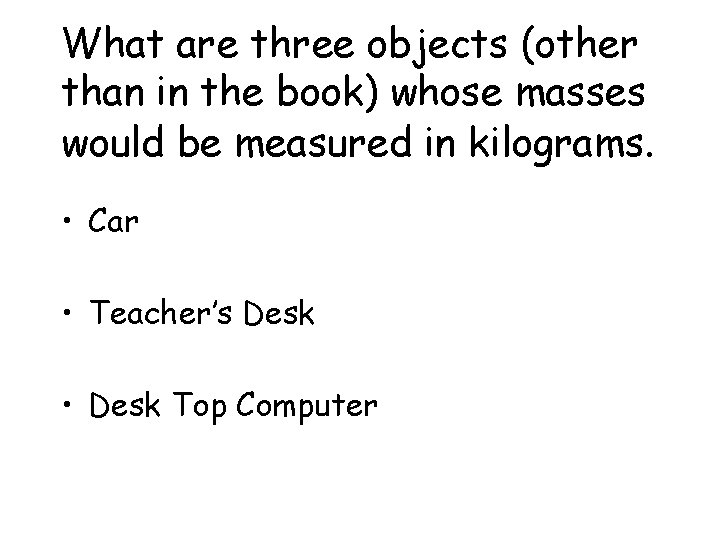What are three objects (other than in the book) whose masses would be measured in kilograms. • Car • Teacher’s Desk • Desk Top Computer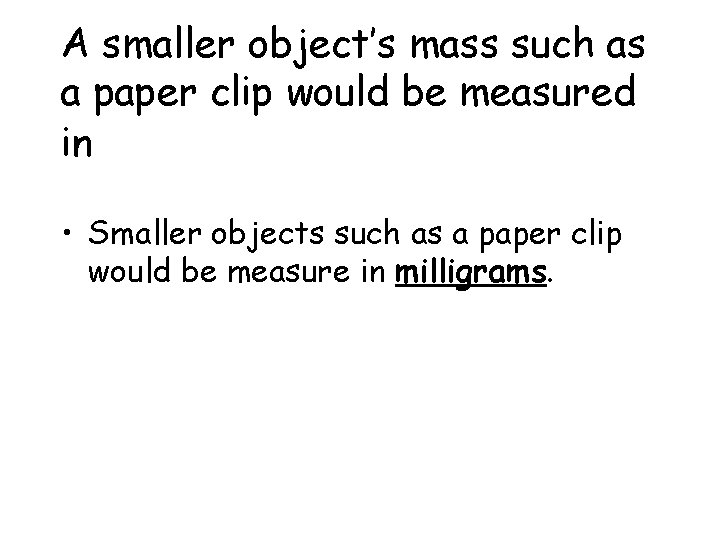A smaller object’s mass such as a paper clip would be measured in • Smaller objects such as a paper clip would be measure in milligrams.Conversions 1 kg = g 1 g = mgConversions 1 kg = 1, 000 g mg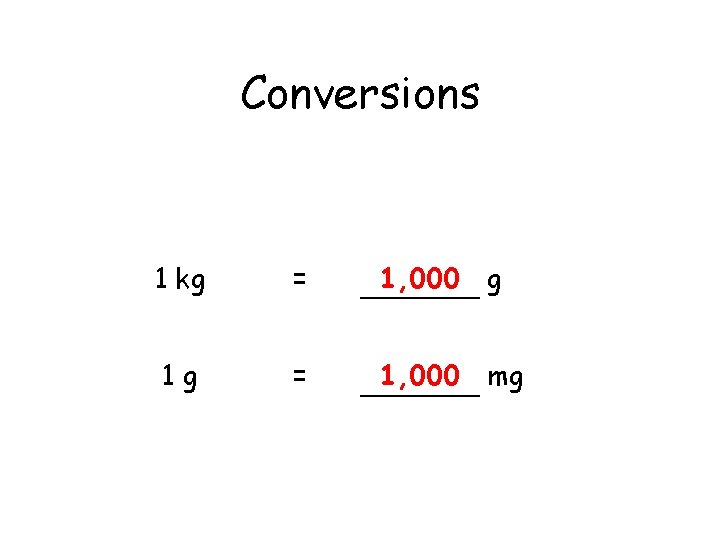Conversions 1 kg = 1, 000 g 1 g = 1, 000 mg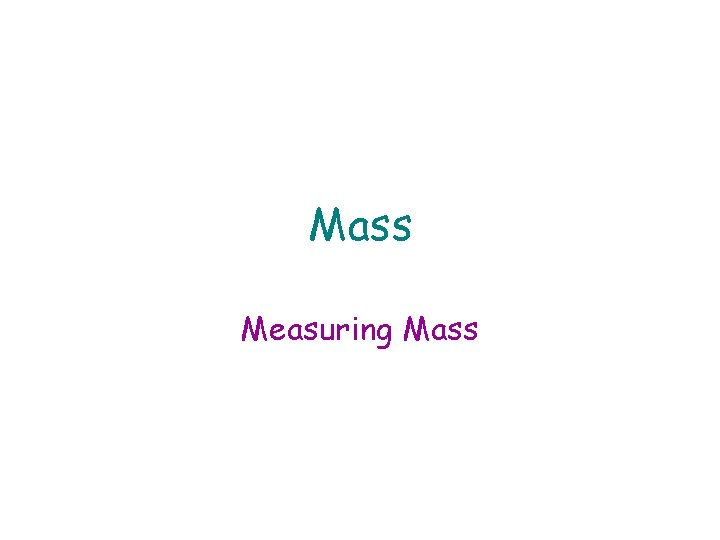Mass Measuring MassHow does a triple-beam balance work? • • A triple-beam balance works by comparing the mass of the object you are measuring to a known mass When you use a triple-beam balance, you: 1. Place the object on the pan 2. Shift the riders on the beams until them balance the mass of the object • • • Start with the large rider (increments of 100) Next, the medium sized rider (increments of 10) Lastly, the small rider (increments of 1)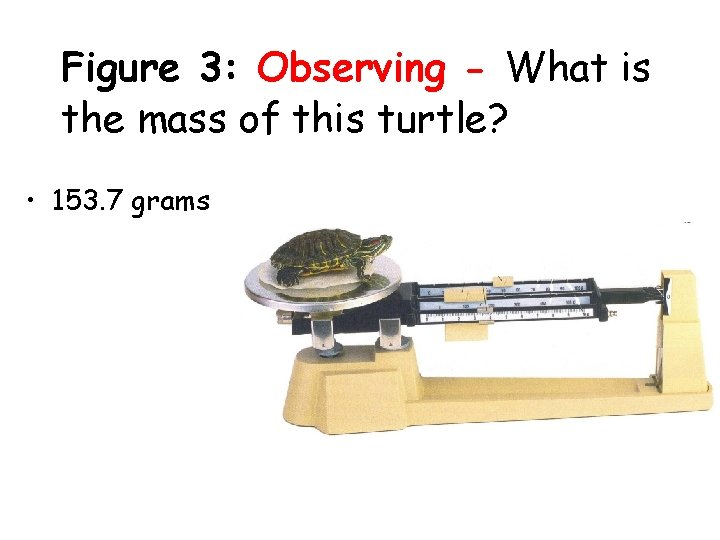Figure 3: Observing - What is the mass of this turtle? • 153. 7 grams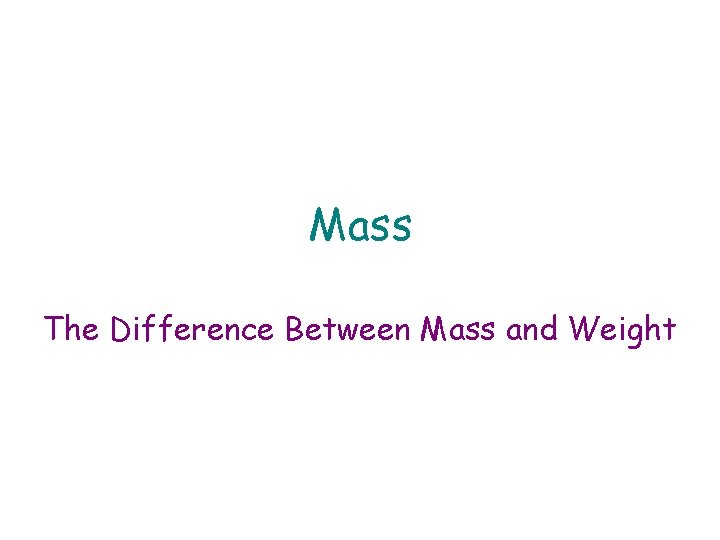Mass The Difference Between Mass and Weight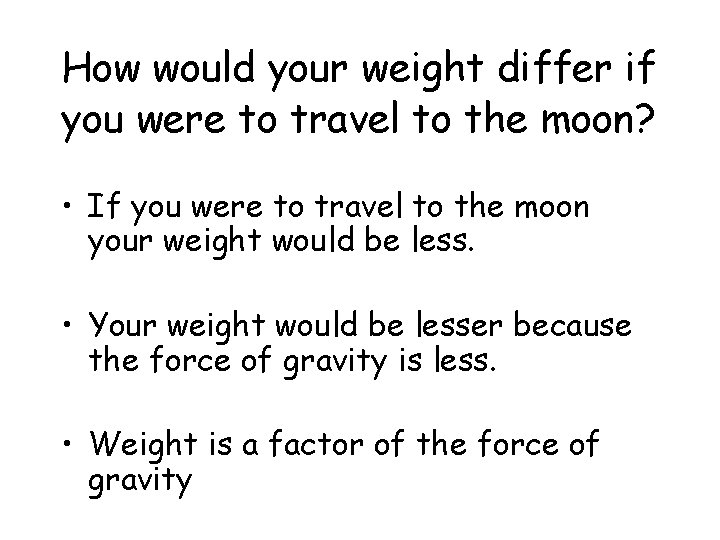How would your weight differ if you were to travel to the moon? • If you were to travel to the moon your weight would be less. • Your weight would be lesser because the force of gravity is less. • Weight is a factor of the force of gravityWhy would your mass stay constant if you were to travel to the moon? • Your mass would stay the same if you were to travel to the moon because mass is the amount of matter that an object contains • Since you are still made up of the same amount of “stuff”, your mass would not change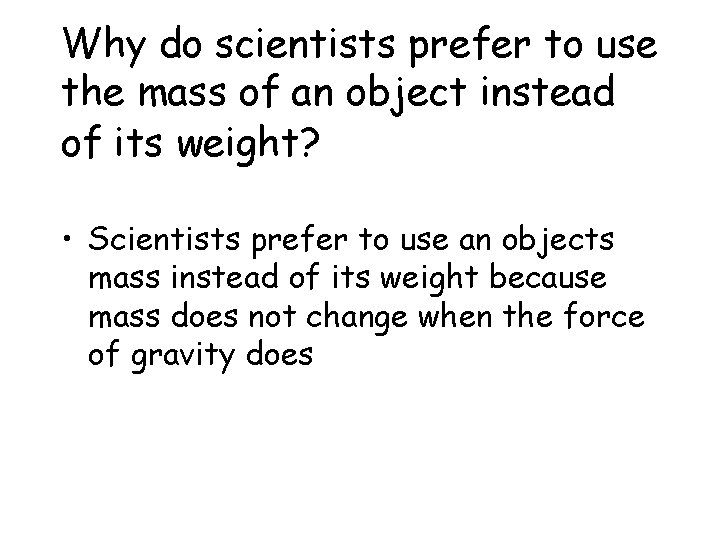Why do scientists prefer to use the mass of an object instead of its weight? • Scientists prefer to use an objects mass instead of its weight because mass does not change when the force of gravity does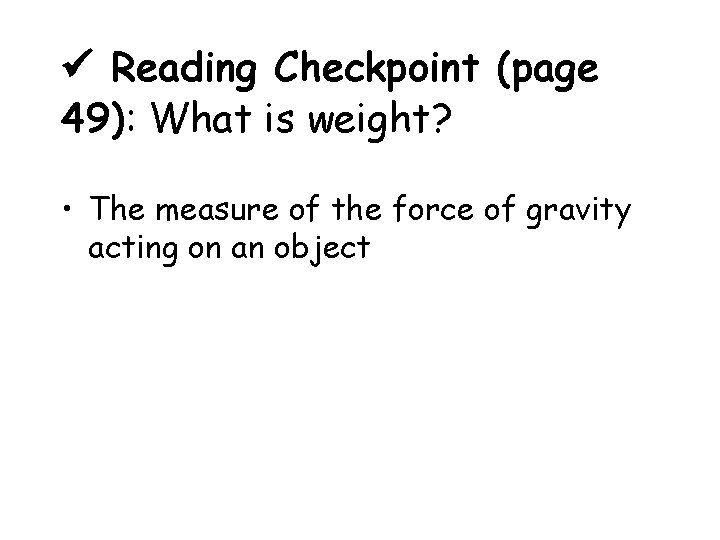Reading Checkpoint (page 49): What is weight? • The measure of the force of gravity acting on an object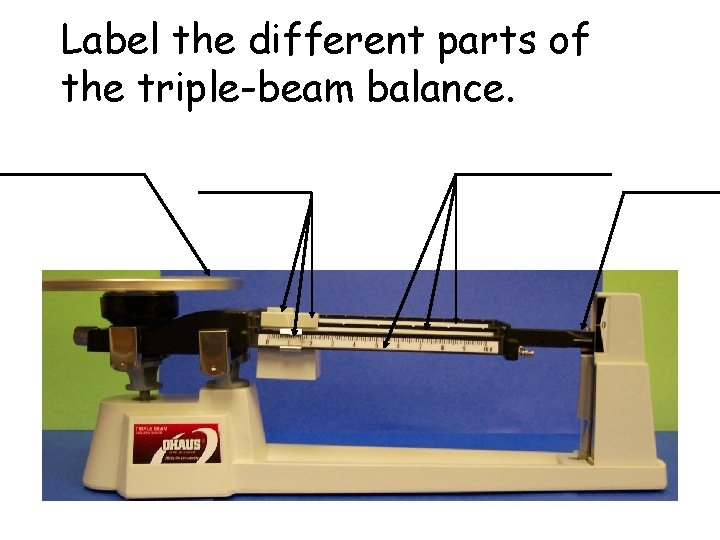Label the different parts of the triple-beam balance.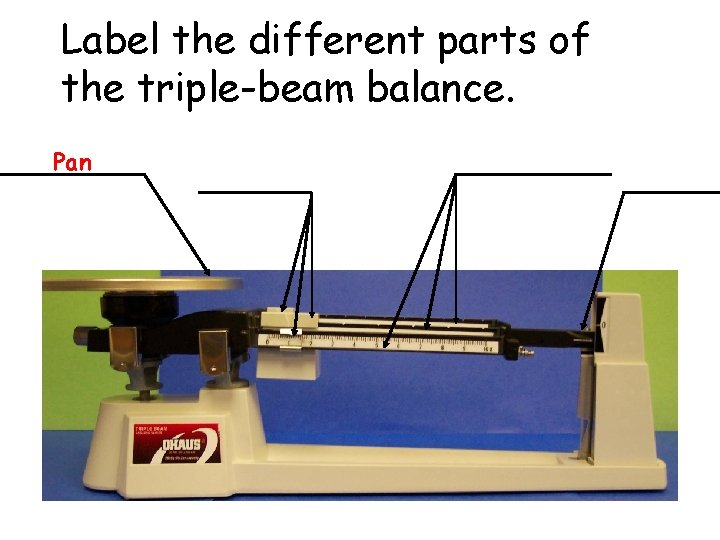Label the different parts of the triple-beam balance. Pan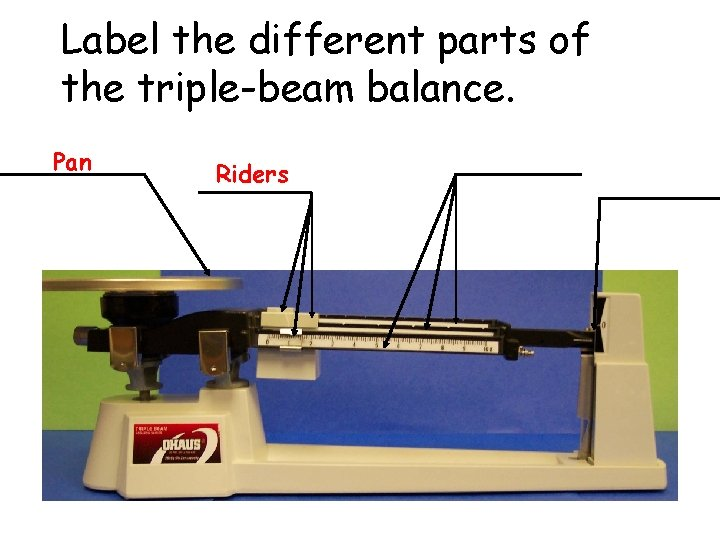Label the different parts of the triple-beam balance. Pan RidersLabel the different parts of the triple-beam balance. Pan Riders Beams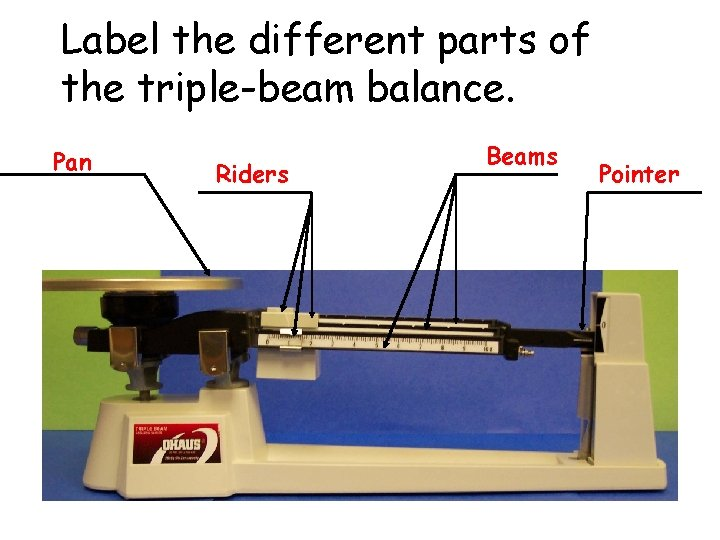Label the different parts of the triple-beam balance. Pan Riders Beams Pointer